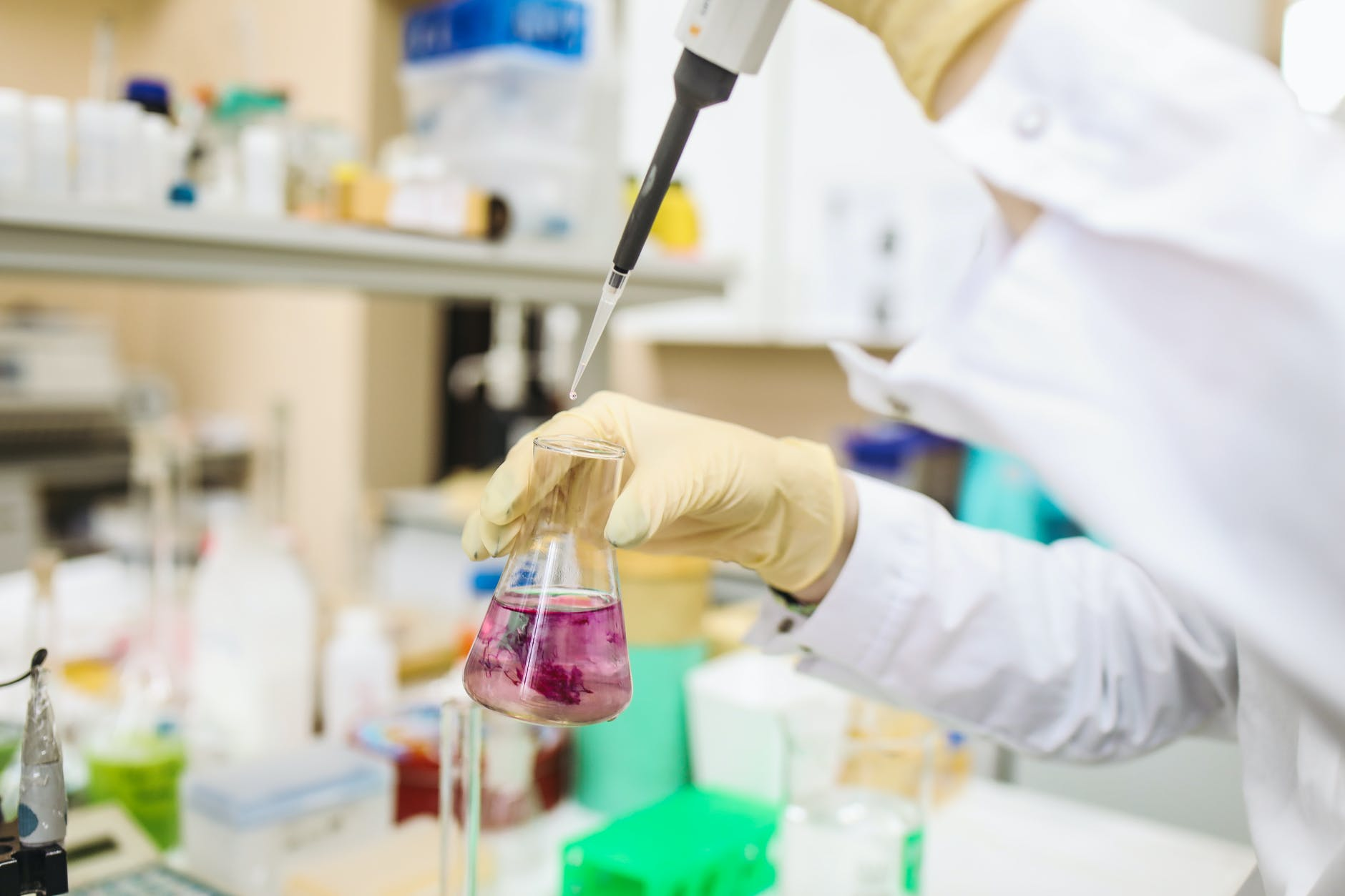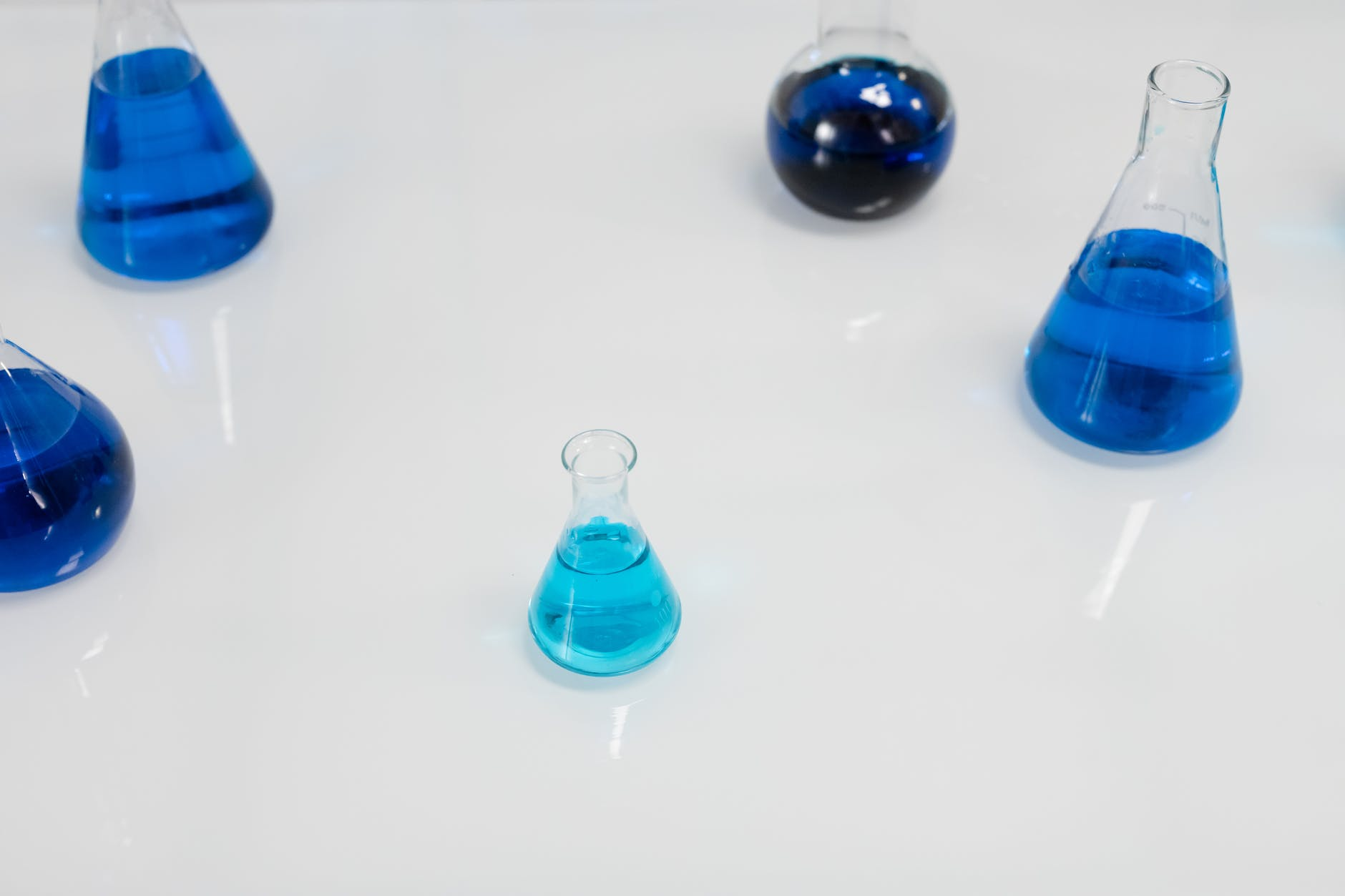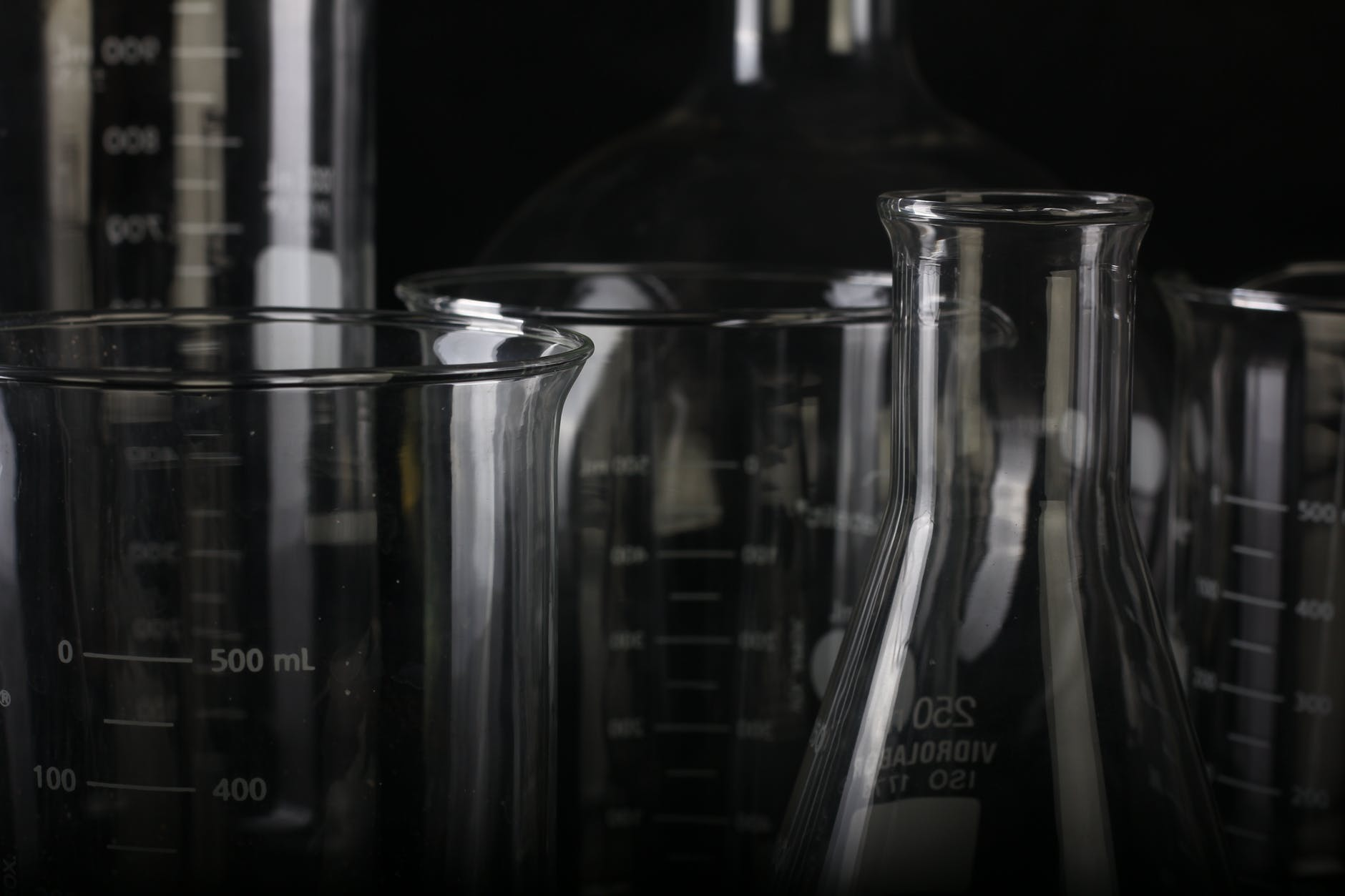# Category Archives: Science# Moles in Chemistry

In this post we will have a brief introduction to moles in chemistry. This fundamental concepts is a part of Stoichiometry which is another important aspect of chemistry.

In chemistry an atomic mass unit (amu) is the mass of a proton or neutron in an atom. This number has been calculated to be.

1.66 X 10-24 g

Knowing this number we can calculate how much a single atom weighs. For example, if we want to calculate the weight in grams of oxygen, we know know that helium has an atomic weight of 4. This means that

The amu cancel each other. This number becomes important because if we take the amu of 1 atom of helium (or any element) in grams and divide by the mass of one atom in grams we get the following number no mattter which element we use.

This number above is how many atoms in 4 grams of helium. This number is called Avogadro’s constant but it also referred to as a mole. Knowing this value, it is possible to calculate the mass of single mole of a molecule. For example, if we want to know the mass of a single mole of glucose we would calculate the amu as shown below.

The mass is as follows

This output tell us that one mole of glucose is 180.18 grams. We can use this information in other ways such as determining how many moles are in a certain number of grams of a substance. If we have 15 grams of magnesium chloride MgCl2. We can calculate how many moles are in this substance as shown below

Step 1 Calculate the amu of the molecule

Mass of MgCl2 = 24.31 amu + 2 * (35.45 amu) = 95.21 amu

Step 2 Determine Conversion Reltionship

1 Mole of MgCl2 = 95.21 grams MgCl2

Step 3 Convert from grams to Moles

We now know that there are about 0.158 moles in 15 grams of magnesium chloride. But we could take this a step further by determining how many molecules are in 15 grams of magnesium chloride as shown below

0.158 * 6.02 * 1023 = 9.84 * 1022

The first number is the number of moles in 15 grams of magnesium chloride and and the second number is one mole.

There are many variations on the calculations that were done here but this is enough to serve as an introduction.# Chemical Equations

Chemical reactions involves the rearrangement of atoms to beget new chemicals. Often these reactions are captured succinctly in what is called a chemical equation. For example, if we want to show how carbon reacts with oxygen to make carbon dioxide we would write the follow chemical equation.

The plus sign means “reacts with” and the arrow means “to make”. Therefore, we can write this chemical equation in English by saying

Carbon reacts with oxygen to make carbon dioxide

Chemical equations need to balance. If you look at the example above, there are the same number of atoms for each element on each side. The example above is rather simple, however, sometimes it is a little trickeier to tell if an chemical equation is balanced.

In the reaction above we have to look carefully to see if the chemical equation is balanced. Starting on the left we have 1 carbon and 4 hydrogens. Next, there is a 2 which means that we multipl everything by 2 that is next to it. In other words, we do not have 2 oxygen atoms but rather 4 (2 x 2 = 4). After the arrow, we have 1 carbon and 2 oxygen atoms and after the plus sign we have 4 hydrogen atoms (2 x 2 = 4) and 2 oxygen (2 x 1 = 2). If we line everything up you can see that this equation is balanced.

There are times when you need to balance a chemical equation. This can get really challlenging but we will do a simple example below.

The chemical equation above is not balance as you can see below

The table above is one process in balancing an equation. We need both sides to equal each other and the simplest way to do this is to multiple the right side by two and we get the following table.

Below is what our balanced chemical equation looks like.

As mentioned previous, placing the 2 in front of the molecule means multiply everything by 2. Such an example like this is really simple but provides a basic understanding of this process.

Conclusion

Chemical equations can be really fun to deal with once you understand how this works. In the beginning, it can be truly frustrating but perseverance will make the difference.# Physical & Chemical Changes in Chemistry

In this post, we will focus most of our attention on physical changes in chemistry with a brief look at chemical changes.

Changes

Physical change is a change to a substance that does not alter the chemical composition. For example, boiling water is a physical change. Generally, physical changes are easy to reverse, such as when steam is cooled to become liquid water.

Chemical change is a change that alters the chemical composition of a substance. An example would be various forms of cooking, such as frying potatoes to make french fries. Unlike physical changes, chemical changes are much harder to reverse. Just as it is impossible to turn french fries back into raw potatoes.

A specific type of physical change is called phase change. There are several different types of phase changes, as listed below.

• melting
• vaporizing
• freezing
• condensing
• sublimation

Many of these are obvious, but they will be explained for clarity. Melting involves a substance moving from a solid to a liquid. Vaporizing takes place as a substance moves from liquid to gas. A substance that moves from a gas to a liquid is called condensing. Freezing is the process of a liquid becoming a solid. Sublimation is a solid moving straight to a gas.

The first four-phase changes are commonly seen in water. Ice melts to become liquid water, water boils/evaporates (vaporizes) to become steam. Water freezes to become ice; in the early morning, it is common in many places to see water on plants due to condensation. Sublimation is tricker to see on a day-to-day basis. The most common example involves carbon dioxide, aka dry ice, which is a favorite tool for Halloween. Other substances that sublimate include arsenic, iodine, and naphthalene (used for mothballs).

Phase changes are related to the kinetic theory of matter, which we will now turn our attention to.

Kinetic Theory of Matter

The kinetic theory of matter states that Molceults have space between them and are in constant random motion. We can say that the more heat, the faster the motion because more energy is present. For solid, the molecules can vibrate, but that is essentially it. All solids are vibrating, such as tables, chairs, desks, etc. However, the vibration is random, and thus the vibrations cancel each other.

Liquids can clearly move about, and this is why they cannot keep a single shape but is formed by their circumstances. This also applies to gasses. The real difference between the various phases is the space around molecules and the speed at which they are moving. When energy is added, molecules move apart and move faster. This explains a solid becoming a liquid and a liquid a gas.

Water breaks many rules in relation to the Kinetic theory of matter. When water freezes, instead of the molecules getting closer together, they actually push out and are thus less dense than water. This is one reason why ice floats and why you would find frozen ice on the top of a lake. The ice floats to the top, and by being on top, it insulates the animals inside the lake from the cold above.

Conclusion

Physical changes play a major role in all of our lives. The phase changes of water are used for various purposes in everyday life. It is beneficial to understand these concepts as they are so commonly encountered.

# Lewis Structures with LaTeX VIDEO

Lewis structures are used to illustrate the valence electrons of an atom. The video below explains how to make these diagrams using LaTeX. For chemistry students this is a great intro to using LaTeX for completing assignments.# Dalton’s Atomic Theory

John Dalton was an 18th-century scientist who made several significant contributions to his field. One of his most prominent works was his Atomic theory. Dalton’s Atomic theory is a major concept in the study of chemistry. In this post, we will look at this theory and share some of the misunderstandings that Dalton had at his time.

Atomic Theory

Dalton’s Atomic Theory has four propositions to it.

1. All matter is made of atoms that cannot be divided or destroyed
2. All atoms of an element are identical in all their properties
3. Compounds are formed by a combo of two or more different kinds of atoms
4. A chemical reaction is a rearrangement of the atoms in the substance

There is little to explain here. Part one states that atoms cannot be divided or destroyed. In other words, the atom is the fundamental unit of the universe. Part 2 states that all atoms are identical in their properties, which implies that every atom of an element has the same number of protons, neutrons, and electrons.

The third component states that compounds are formed by two or more different atoms. For example, one compound would be H2O which is water. Since there are two elements in H2O, it meets the definition of a compound. We also call such a compound a molecule. Component four states that a chemical reaction is a rearrangement of the atoms in the substance. An example of this would be digestion which involves significant chemical changes to the food.

Problems with Dalton’s Theory

Despite the brilliance of Dalton’s theory, several problems have arisen as researchers have continued to explore the mysteries of chemistry. For example, the first proposition of Dalton states that atoms cannot be divided or destroyed. Both of these claims are false. We now know that atoms are made of protons, neutrons, and electrons. In addition, atoms can be destroyed, which happens at any nuclear power plant through fission. Nuclear fission involves neutrons hitting atoms which causes them to split.

Dalton was also incorrect regarding his second proposition about the same atoms having the same properties. With the discovery of the neutron, it became clear that atoms may have the same chemical properties but not the same physical properties. The reason for this is that having a different number of neutrons affects the atom’s weight. When atoms of the same element have different neutrons, we call these isotopes.

Conclusion

Dalton’s work in the study of atoms is something to be praised. It is understandable that perhaps he got some things wrong. The purpose of science is to grow and improve over time, and this means that sometimes great scientists are right, but they must also be wrong.# Terms Related to Matter

Matter is the physical stuff that everything around us is generally made of. Trees, birds, water, etc., are all examples of matter. Since almost everything is considered matter, scientists have naturally found ways to classify matter to better understand it.

Types of Matter

One way matter is classified whether it is a pure substance or a mixture. A pure substance is a substance that has the same properties throughout out it. An example of a pure substance would be salt or sugar. Both of the substances are only made of salt or sugar, and the properties of these two substances are the same if you have one or the other in a sample.

On the other hand, a mixture is a combination of two or more substances. For example, if you have salt and pepper inside the same shaker, this is a mixture. This is because separating the salt and the pepper from each other is possible. Separating pure substances is generally not possible physically. However, pure substances can further be broken down into elements and compounds.

Elements are fundamental substances that cannot be broken down into simpler substances. The periodic table contains all known elements. Examples include oxygen, sodium, carbon, etc. Compounds are pure substances that are made of two or more elements. Compound examples include salt, sugar, carbon dioxide, etc.

More on Mixtures

Returning to mixtures, there are two types of mixtures: homogenous and heterogeneous. Homogenous mixtures have the same composition throughout the sample. Examples include milk and sugar water. In both of these examples, the substances that make up the mixture are evenly spread throughout the sample.

Heterogeneous mixtures have different compositions in parts of the sample. A classic example of this is salad dressing. When salad dressing is allowed to sit, it separates clearly into the various substances/homogenous mixture that it is made up of. This is why salad dressing must be shaken before it is enjoyed.

Law of mass conservation

Antoine Lauren de Lavoisier developed the law of mass conservation, which states that in any chemical or physical process, the total mass of everything involved must remain the same. This means that if you start with 5 kg of wood and burn it, there will still be 5kg of matter in a different form. You might see a pile of ashes that weighs less but what happens is that some of the matter was converted to gases and smoke in the burning process. Essentially, matter can be created or destroyed but can only be converted or broken down.

Conclusion

No pun intended, but matter matters. For students, it is important to develop an understanding of concepts related to chemistry. Doing so may help at least some of them prepare for whatever occupation they may have in the future.